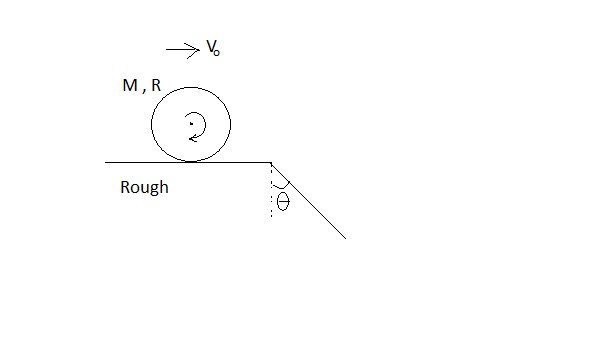# Cylinders don't jump and slip!An uniform solid cylinder of mass $M$ and radius $R$ rolls without slipping on horizontal passing into an inclined plane which makes an angle $\theta$ with the vertical as shown in figure.

Find the maximum value of the velocity ${v}_{o}$ which still permits the cylinder to roll onto the inclined plane section without a jump.

If it's maximum value can be expressed as

${v}_{o_\text{max}} = \sqrt {\cfrac {gR}{a} (b\sin \theta - c)}\text{,}$

then find the value of $a+b+c$.

Details and Assumptions

$\bullet$ There is sufficient friction on the entire surface (with coefficient of friction $\mu$).

$\bullet$ Here $a, b, c$ are positive integers such that $\text{gcd}(a, b, c)=1$.

Source : I solved This question somewhere else ! And Liked it So I share this !

×